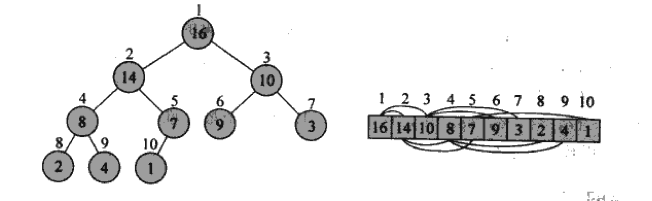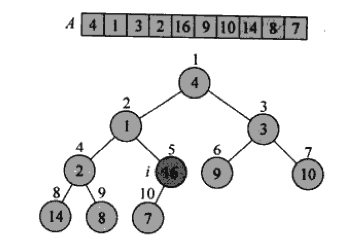# 堆排序实现

2014/02/26 17:15

## 堆排序实现：• 那么对于一个普通的数组，我们要怎么让其变成最大堆呢，比如：/**
* 最大堆化数组arr[index]为根的子树
* @param arr 对应数组
* @param index 根索引， 0 ～ arr.length－1
* @param lastIndex 比较操作的最大索引
*/
private static void maxHeapify(int arr[], int index, int lastIndex){
int leftChildIndex = left(index);
int rightChildIndex = right(index);

int biggest = index;
// 若果左边的孩子更大
if ((leftChildIndex <= lastIndex)
&& arr[index] < arr[leftChildIndex]){
biggest = leftChildIndex;
}
// 若右边的孩子还更大
if ((rightChildIndex <= lastIndex)
&& arr[biggest] < arr[rightChildIndex]){
biggest = rightChildIndex;
}
// 需要进行调整
if (biggest != index){
int temp = arr[biggest];
arr[biggest] = arr[index];
arr[index] = temp;
// 递归最大堆化子树
maxHeapify(arr, biggest, lastIndex);
}
}
// 获取左孩子索引
private static int left(int index) {
return 2*index+1;
}
// 获取右孩子索引
private static int right(int index) {
return 2*index+2;
}

/**
* 对整个数组进行最大堆化:
* 可对每个非叶子节点出发进行最大堆化，
* 	注意叶子节点索引为: [(arr.length)/2，+1, +2...n]，我们没必要对它们进行最大堆化
* 将数组构建为最大堆的线性复杂度为: O(n)
* @param arr 对应数组
*/
private static void buildMaxHeap(int arr[]){
for (int i=(arr.length-1)/2; i>=0; i--){ //必须从大到小
maxHeapify(arr, i, arr.length-1);
}
}

/**
* 堆排序算法：
* 不停交换a到最后
* @param arr 待排序数组
*/
private static void heapSort(int[] arr) {
//将数组最大堆化
buildMaxHeap(arr);
for (int i=arr.length-1; i>0; i--){
arr = arr^arr[i];
arr[i] = arr[i]^arr;
arr = arr^arr[i];
//将第一个(根)继续进行最大堆化,
//但经过上面的交换操作, 会依次排除排除倒数第i-1元素
maxHeapify(arr, 0, i-1);
}
}

int arr[] = new int[]{4, 1, 3, 2, 16, 9, 10, 14, 8, 7};
heapSort(arr);### 作者的其它热门文章

0
10 收藏

0 评论
10 收藏
0